# Multiple Choice Questions Chemical Kinetics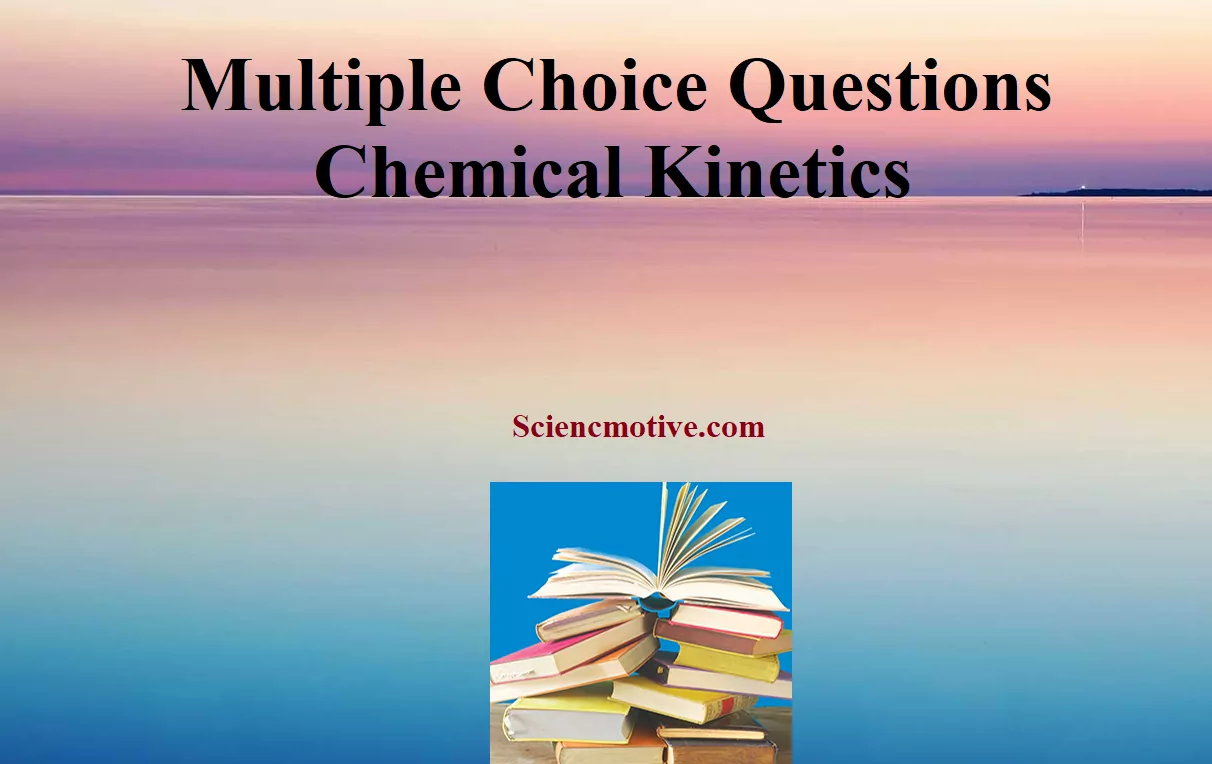Multiple Choice Questions Chemical Kinetics

Que 1. In the reaction 2 NO + Cl2 2 NOCl, it has been found that doubling the concentration of both the reactants increases the rate by a factor of eight but doubling the chlorine concentration alone only doubles that rate. Which of the following statement is correct?
1. The reaction is second order in Cl2
2. The reaction is first order in NO
3. The overall order of the reaction is 2
4. The overall order of the reaction is 3
Ans 1. The overall order of the reaction is 2.

Que 2. The half-life period of a first-order reaction is 100° seconds. Its rate constant is:
(a) 0.693 sec–1
(b) 6.93 × 10–3 sec–1
(c) 6.93 × 10–2 sec–1
(d) None of these
Ans 2. (b).
Explanation: We know half-life for first order is
t1/2 = 0.693/k
Therefore k = 0.693/t1/2
k = 0.693/100 = 6.93 × 10–3 sec–1

Que 3. The term – dc/dt in a rate equation refers to
(a) The concentration of a reactant
(b) The decrease in conc. of the reactant with time
(c) The velocity constant of the reaction
(d) None of these
Ans 3. (b) The decrease in conc. of the reactant with time

Que 4. . The role of a catalyst is to change
(i) Gibbs energy of the reaction.
(ii) Enthalpy of reaction.
(iii) Activation energy of the reaction.
(iv) Equilibrium constant.
Ans 4. Activation energy of reaction

Que 5. What is the activation energy for the reverse of this reaction? N2 + O4(g) 2NO2(g) Data for the given reaction is ∆H = 54 KJ/mol and Ea = 57.2 KJ.
(a) – 54 KJ
(b) 3.2 KJ
(c) 60.2 KJ
(d) 111.2 KJ
Ans 5. (b) 3.2 KJ

Multiple Choice Questions Chemical Kinetics

Que 6. The dimensions of the rate constant of a second-order reaction involve:
(a) Time and Concentration
(b) Neither time nor Concentration
(c) Time only
(d) Concentration only
Ans 6. (a) Time and Concentration
Explanation: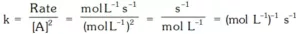Que 7. Which of the following best explains the effects of a catalyst on the rate of a reversible reaction?
(a) It decreases the rate of the reverse reaction
(b) It increases the kinetic energy of the reacting molecules
(c) It moves the equilibrium position to the right
(d) It provides a new reaction path with lower activation energy
Ans 7. Catalyst decreases the activation energy of the system.

Que 8. Which of the following statements is not correct about the order of a reaction?
(i) The order of a reaction can be a fractional number.
(ii) Order of a reaction is experimentally determined quantity.
(iii) The order of a reaction is always equal to the sum of the stoichiometric coefficients of reactants in the balanced chemical equation for a reaction.
(iv) The order of a reaction is the sum of the powers of the molar concentration of the reactants in the rate law expression.
Ans 8. (iii) The order of a reaction is always equal to the sum of the stoichiometric coefficients of reactants in the balanced chemical equation for a reaction.

Que 9. The rate constant of a reaction becomes equal to the pre-exponential factor when:
(a) The absolute temperature is zero
(b) The activation energy is infinity
(c) The absolute temperature is infinity
(d) The activation energy is zero
Ans 9. (c) The absolute temperature is infinity

Que 10. In the reaction, 2A + B A2B if the concentration of A is doubled and that of B is halved, then the rate of the reaction will:
(a) Increase 2 times
(b) Increase 4 times
(c) Decrease 2 times
(d) Remain the same
Ans 10. (a)
Explanation: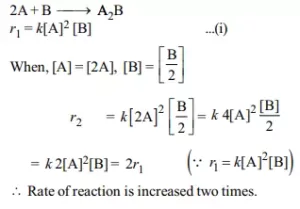Multiple Choice Questions Chemical Kinetics

Que 11. Which of the following statements is incorrect about the collision theory of chemical reaction?
(i) It considers reacting molecules or atoms to be hard spheres and ignores their structural features.
(ii) Number of effective collisions determines the rate of reaction.
(iii) Collision of atoms or molecules possessing sufficient threshold energy results into the product formation.
(iv) Molecules should collide with sufficient threshold energy and proper orientation for the collision to be effective.
Ans 11. (iii) Collision of atoms or molecules possessing sufficient threshold energy results in the product formation.

Que 12. The initial rate of zero-order reaction of the gaseous reaction A(g) 2B(g) is 10–2 M min–1. If the initial concentration of A is 0.1M. What would be the concentration of B after 60 sec?
(A) 0.09 M
(B) 0.01 M
(C) 0.02 M
(D) 0.002 M
Ans 12. (C).
Explanation: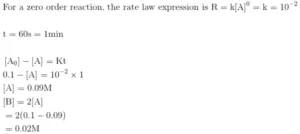Multiple Choice Questions Chemical Kinetics

Que 13. Select the rate law that corresponds to the data shown for the following reaction:
A + B → C

 Expt. No. (A) (B) Initial rate 1 0.012 0.035 0.10 2 0.024 0.070 0.80 3 0.024 0.035 0.10 4 0.012 0.070 0.80

(a) Rate = k[B]3
(b) Rate = k [B]4
(c) Rate = k [A] [B]3
(d) Rate = k [A]2 [B]2
Ans 13. (a)
Explanation: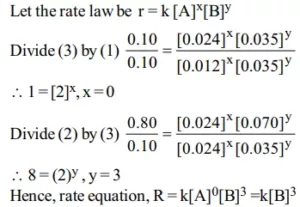Que 14. In a pseudo-first-order reaction of hydrolysis of an ester in H2O, the following results were obtained:

 t/s 0 30 60 90 Ester M/L 0.55 0.31 0.17 0.085

Calculate the pseudo first-order rate constant for the hydrolysis of the ester.
(a) 3.08 × 10-2 sec-1
(b) 1.1 × 103 sec-1
(b) 2.98 × 102 sec-1
(d) 1.98 × 10-2 sec-1
Ans 14. (d) 1.98 × 10-2 sec-1
Explanation: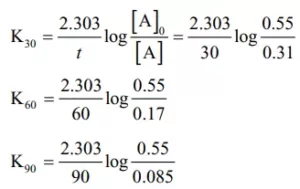K = 1.98 × 10-2 sec-1

Que 15. Which of the following statements is correct?
(i) The rate of a reaction decreases with the passage of time as the concentration of reactants decreases.
(ii) The rate of a reaction is the same at any time during the reaction.
(iii) The rate of a reaction is independent of temperature change.
(iv) The rate of a reaction decreases with an increase in the concentration of reactant(s).
Ans 15. (i) The rate of a reaction decreases with the passage of time as the concentration of reactants decreases.

Multiple Choice Questions Chemical Kinetics